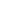# Is 0( zero) a multiple of 10?

a) Yes
b) No
c) Absurd
d) Ambiguous

 Yes. An integer a is a multiple of an integer b means that ab=integer: so, as 0 divided by any integer (except zero itself) yields an integer then yes, zero is a multiple of every integer (except zero itself).
Share :
+
Next
« Prev
Prev
Next »